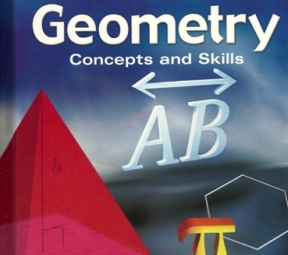M A T H   T U T O R I N G
ONE-ON-ONE
TEST PREPARATION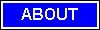Geometry is one of the oldest mathematical sciences. Initially a body of practical knowledge concerning lengths, areas, and volumes, in the 3rd century BC geometry was put into an axiomatic form by Euclid, whose treatment-Euclidean geometry-set a standard for many centuries to follow. The introduction of coordinates and the concurrent development of algebra marked a new stage for geometry, since geometric figures, such as plane curves, could now be represented analytically, i.e., with functions and equations.The subject of geometry was further enriched by the study of intrinsic structure of geometric objects that originated with Euler and Gauss and led to the creation of topology and differential geometry. Geometry is a branch of mathematics that is concerned with the properties of configurations of geometric objects - points, (straight) lines, and circles being the most basic of these. Although the word geometry derives from the Greek geo (earth) and metron (measure) [Words], which points to its practical roots,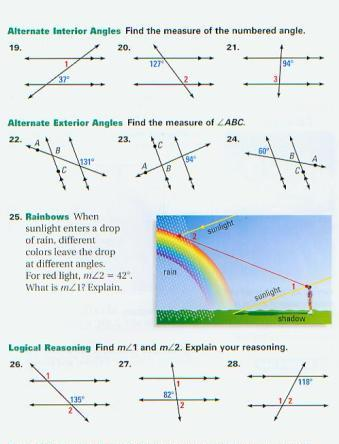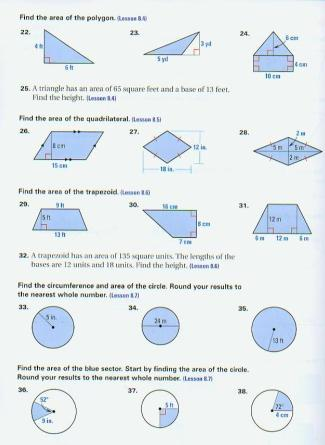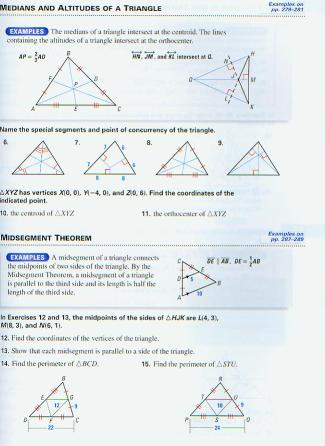sitemap